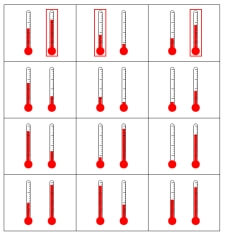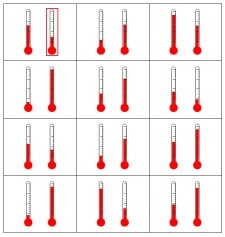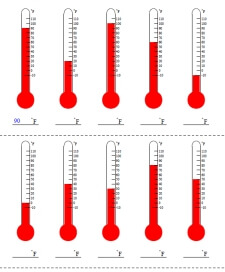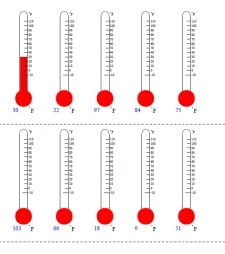Worksheets and No Prep Teaching Resources
Math Worksheets

Temperature Worksheets

Temperature can be a hot topic for students, and it is a subject that can be confusing unless presented in the right way. These expertly-designed, sharp worksheets give students concrete examples that will help them understand Fahrenheit and Celsius clearly. With this kind of practice, students will soon feel that reading thermometers is a cool skill.

Temperature
Is the temperature hot or cold in the picture?
Color the thermometer to show the temperature
Color the thermometer to show the temperature

Temperature: Circle the Warmer or Colder TemperatureCircle the warmer temperature (answers on first row are given) Circle the colder temperature (answers on first row are given) Each page will randomly pick either warmer or colder (answers on first row are given)Circle the warmer temperature Circle the colder temperature Each page will randomly pick either warmer or colder

Temperature: Write the Temperature and Color in the Temperature
FahrenheitFahrenheit: Write the temperature - no negatives and tens only Fahrenheit: Write the temperature - tens only Fahrenheit: Write the temperature - no negatives Fahrenheit: Write the temperatureFahrenheit: Color in the temperature - no negatives and tens only Fahrenheit: Color in the temperature - tens only Fahrenheit: Color in the temperature - no negatives Fahrenheit: Color in the temperature

CelsiusCelsius: Write the temperature - no negatives and tens only Celsius: Write the temperature - tens only Celsius: Write the temperature - no negatives Celsius: Write the temperatureCelsius: Color in the temperature - no negatives and tens only Celsius: Color in the temperature - tens only Celsius: Color in the temperature - no negatives Celsius: Color in the temperature

Fahrenheit or Celsius (randomly picked mix)Write the temperature - no negatives and tens only Write the temperature - tens only Write the temperature - no negatives Write the temperatureColor in the temperature - no negatives and tens only Color in the temperature - tens only Color in the temperature - no negatives Color in the temperature

Measurement Logic Puzzles
High and low temperatures

Temperature and Scientific Notation
Temperature Conversions
Scientific Notation

Metric System: Problem Solving Puzzles
Temperatures

Metric System: Metric System Crossword Number Puzzles
Convert Between Fahrenheit and Celsius

Measurement

Have a suggestion or would like to leave feedback?